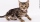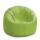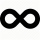# Fraction calculator

The calculator performs basic and advanced operations with fractions, expressions with fractions combined with integers, decimals, and mixed numbers. It also shows detailed step-by-step information about the fraction calculation procedure. Solve problems with two, three, or more fractions and numbers in one expression.

## Result:

### (5/6) : (2/3) = 5/4 = 1 1/4 = 1.25

Spelled result in words is five quarters (or one and one quarter).

### How do you solve fractions step by step?

1. Divide: 5/6 : 2/3 = 5/6 · 3/2 = 5 · 3/6 · 2 = 15/12 = 3 · 5 /3 · 4 = 5/4
Dividing two fractions is the same as multiplying the first fraction by the reciprocal value of the second fraction. The first sub-step is to find the reciprocal (reverse the numerator and denominator, reciprocal of 2/3 is 3/2) of the second fraction. Next, multiply the two numerators. Then, multiply the two denominators. In the following intermediate step, cancel by a common factor of 3 gives 5/4.
In other words - five sixths divided by two thirds = five quarters.

#### Rules for expressions with fractions:

Fractions - simply use a forward slash between the numerator and denominator, i.e., for five-hundredths, enter 5/100. If you are using mixed numbers, be sure to leave a single space between the whole and fraction part.
The slash separates the numerator (number above a fraction line) and denominator (number below).

Mixed numerals (mixed fractions or mixed numbers) write as integer separated by one space and fraction i.e., 1 2/3 (having the same sign). An example of a negative mixed fraction: -5 1/2.
Because slash is both signs for fraction line and division, we recommended use colon (:) as the operator of division fractions i.e., 1/2 : 3.

Decimals (decimal numbers) enter with a decimal point . and they are automatically converted to fractions - i.e. 1.45.

The colon : and slash / is the symbol of division. Can be used to divide mixed numbers 1 2/3 : 4 3/8 or can be used for write complex fractions i.e. 1/2 : 1/3.
An asterisk * or × is the symbol for multiplication.
Plus + is addition, minus sign - is subtraction and ()[] is mathematical parentheses.
The exponentiation/power symbol is ^ - for example: (7/8-4/5)^2 = (7/8-4/5)2

#### Examples:

subtracting fractions: 2/3 - 1/2
multiplying fractions: 7/8 * 3/9
dividing Fractions: 1/2 : 3/4
exponentiation of fraction: 3/5^3
fractional exponents: 16 ^ 1/2
adding fractions and mixed numbers: 8/5 + 6 2/7
dividing integer and fraction: 5 ÷ 1/2
complex fractions: 5/8 : 2 2/3
decimal to fraction: 0.625
Fraction to Decimal: 1/4
Fraction to Percent: 1/8 %
comparing fractions: 1/4 2/3
multiplying a fraction by a whole number: 6 * 3/4
square root of a fraction: sqrt(1/16)
reducing or simplifying the fraction (simplification) - dividing the numerator and denominator of a fraction by the same non-zero number - equivalent fraction: 4/22
expression with brackets: 1/3 * (1/2 - 3 3/8)
compound fraction: 3/4 of 5/7
fractions multiple: 2/3 of 3/5
divide to find the quotient: 3/5 ÷ 2/3

The calculator follows well-known rules for order of operations. The most common mnemonics for remembering this order of operations are:
PEMDAS - Parentheses, Exponents, Multiplication, Division, Addition, Subtraction.
BEDMAS - Brackets, Exponents, Division, Multiplication, Addition, Subtraction
BODMAS - Brackets, Of or Order, Division, Multiplication, Addition, Subtraction.
GEMDAS - Grouping Symbols - brackets (){}, Exponents, Multiplication, Division, Addition, Subtraction.
Be careful, always do multiplication and division before addition and subtraction. Some operators (+ and -) and (* and /) has the same priority and then must evaluate from left to right.

## Fractions in word problems:

• Double division0.25 divided by 1/2 divided by 14
• Cat foodAnna has 3/4 bag of cat food. Her cat eats 1/10 of a bag per day. How many days will food last?
• Equivalent expressionsA coach took his team out for pizza after their last game. There were 14 players, so they had to sit in smaller groups at different tables. Six players sat at one table and got 4 small pizzas to share equally. The other players sat at the different table
• Cutting wireIf you cut a 3 ½ ft length wire into pieces that are 2 inches long, how many pieces of wire will you have?
• Penny coinsOne 2p coin is 1/8 inch thick. A pile of 2p is 1 1/2 inch high. How many coin are there in the pile
• A servingA serving of rice is 1/2 of a cup. How many servings are there in a 3 1/2-cup bag of rice?
• Two numbers and its productThe product of two numbers are 2/3. If on of them is 1/10, what is the other?
• The length 6The length of 12 pipes is 10 1/2 meters. (1) find the length of one pipe (2) also find the length of 7 pipes
• An airplaneAn airplane flies 1440 km in 2 1/4 hours. What is its average speed in km per hour?
• Percent calculationCalculate 8% if 44% is 32.
• EvaluateThe division of numbers 6 and 3 increase by-product of the numbers 115 and 0.1
• Division by zeroFraction 5 by 2. if 3 is added to numerator and 2 is subtracted from the denominator then the new fraction is:
• Numbers divisionWith what number should be divided mixed number 2 3/4 to get 11/12?